# Data Lab Visualizations Explained

In the impact.com Data Lab, you can present your data in 12 visualizations. These all present data in different ways: some visualizations require both Dimensions and Measures, and some only require Measures.

## Data Table

The Data Table will fill with the given Dimensions and Measures you select from the right toolbar. Dimensions will fill the rows, and Measures will fill the columns. Filters can be applied to the entire table.

#### Note

This is the only visualization that can display action-level Dimensions. If you include action-level data in your table, some fields will become unavailable to use in the same table. Unavailable Dimensions will be grayed out in the right toolbar.## Line

The Line Chart will use Dimensions to fill data points on the x-axis and Measures (called Series when populating data in the right toolbar) to fill points on the y-axis. Multiple lines can exist if you have multiple Dimensions or multiple Measures. Filters will be applied to the entire chart.## Area

The Area Chart will use Dimensions to fill data points on the x-axis and Measures to fill points on the y-axis. Multiple areas can exist if you have multiple Measures. Filters can be applied to the entire chart.## Treemap

The Treemap will use Dimensions to differentiate the groups that will appear in the visualization and Measures to determine the groups' values (sizes) relative to each other. Filters can be applied to the entire chart.## Vertical Bar

The Vertical Bar Chart will use Dimensions to fill data points on the x-axis and Measures to fill points on the y-axis. Multiple bars can exist if you have multiple Dimensions. Filters can be applied to the entire chart.## Vertical Stack

The Stacked Bar Chart will use Dimensions to fill data points on the x-axis and Measures to fill points on the y-axis. Bars will stack according to the Measures you select. Filters can be applied to the entire chart.## Horizontal Bar

The Horizontal Bar Chart will use Dimensions to fill data points on the y-axis and Measures to fill points on the x-axis. Multiple bars can exist if you have multiple Dimensions. Filters can be applied to the entire chart.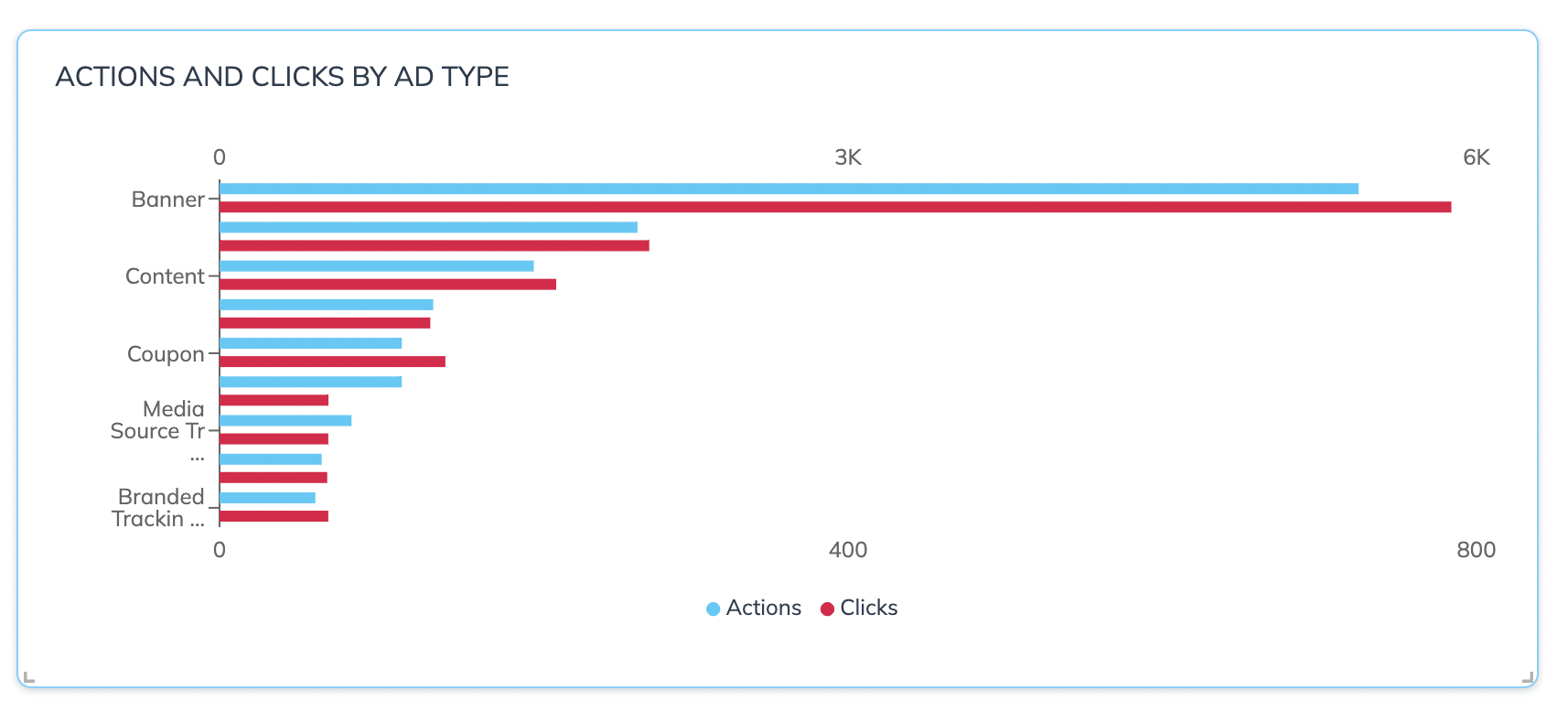## Horizontal Stack

The Horizontal Stack Chart will use Dimensions to fill data points on the y-axis and Measures to fill points on the x-axis. Bars stack according to the Measures you select. Filters can be applied to the entire chart.## Funnel

The Funnel shows where the majority of your value (represented by a Measure) comes from, separated by groups (represented by a Dimension). For example, you can use this visualization to see which partners bring you the most revenue or which event type is driving the most actions.## Donut

The Donut shows where the majority of your value (represented by a Measure) comes from, separated by grouping (represented by a Dimension). Use this chart to see how big a portion of the total value a specific group (or Dimension) is driving.## Goals

The Goals Chart will present a gauge of a Measure's progress towards a number goal you provide. Only one Measure can be selected, and a filter can be applied to this visualization.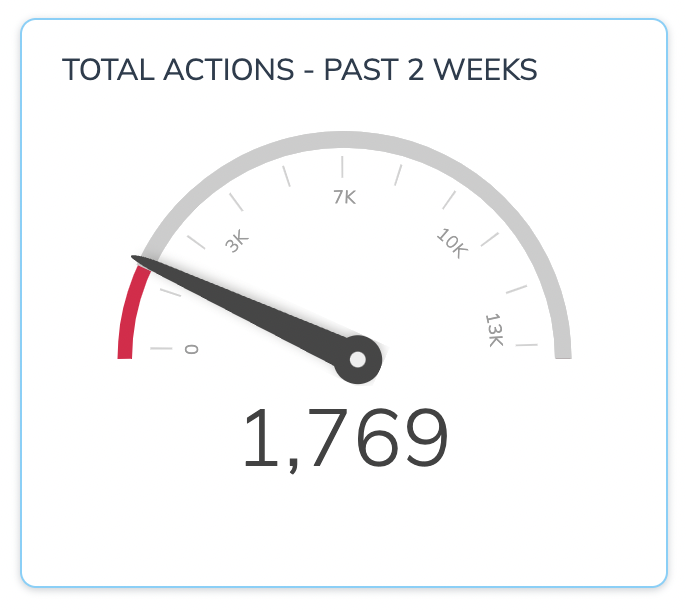## Number

The Number Chart will present the data point of one Measure. A filter can be applied to this number.## Filter visualization data

You can also filter data that appears in your visualization by a specific Dimension (blue icon elements only. e.g., Customer Status is New) or Condition (red icon elements only, e.g., Revenue > \$100).

1. Find the element you want to add from the right-most column.
2. Drag and drop that element under Filter By Dimension or Filter By Condition.
3. Once you've added the minimum required data points for that visualization, the visualization will automatically update to display your data.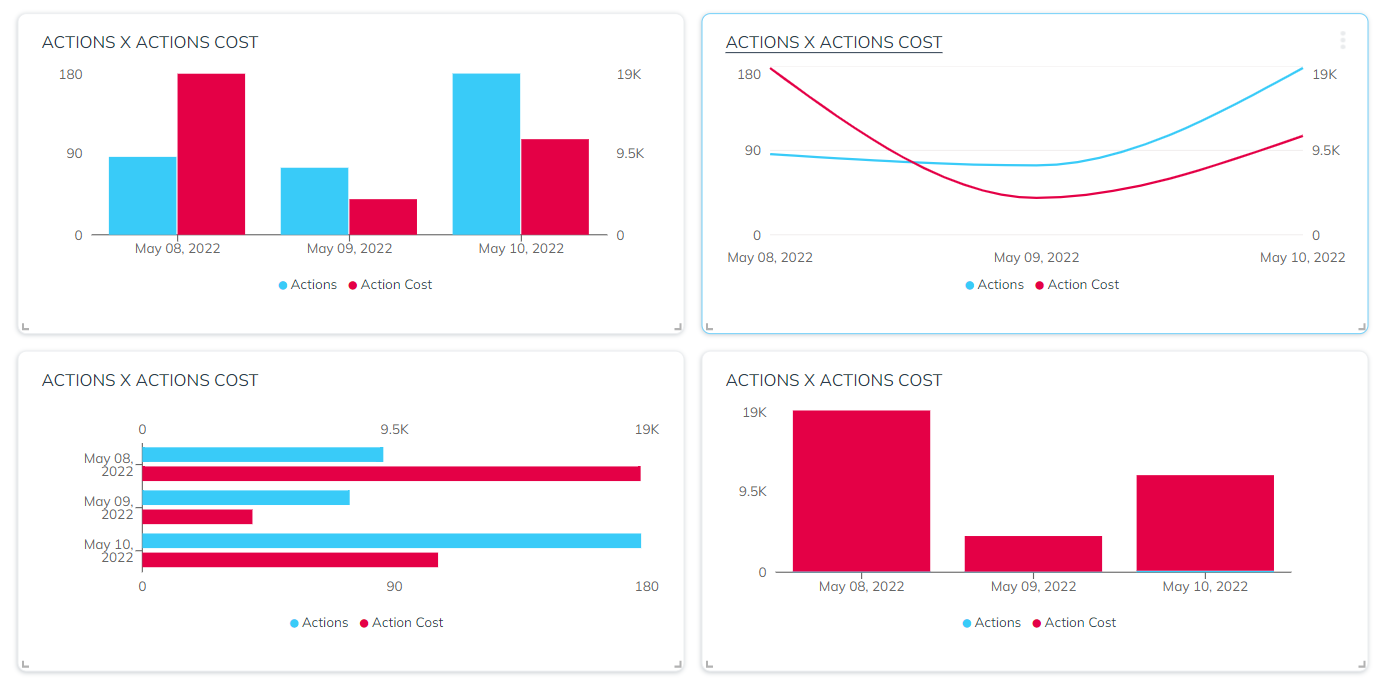## Summarize and compare data point information

You can get a summary of the information in a data point by hovering over that data point in your visualization.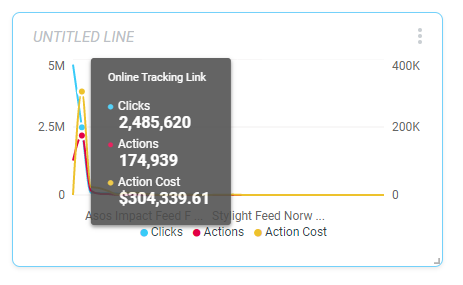When comparing data over two periods of time, you can also sort data table columns by the following:

• Absolute Change (the number difference between the two periods)
• Percentage Change (the percentage difference between the two periods)
• Current (sort by the current date range values)
• Previous (sort by the previous date range values)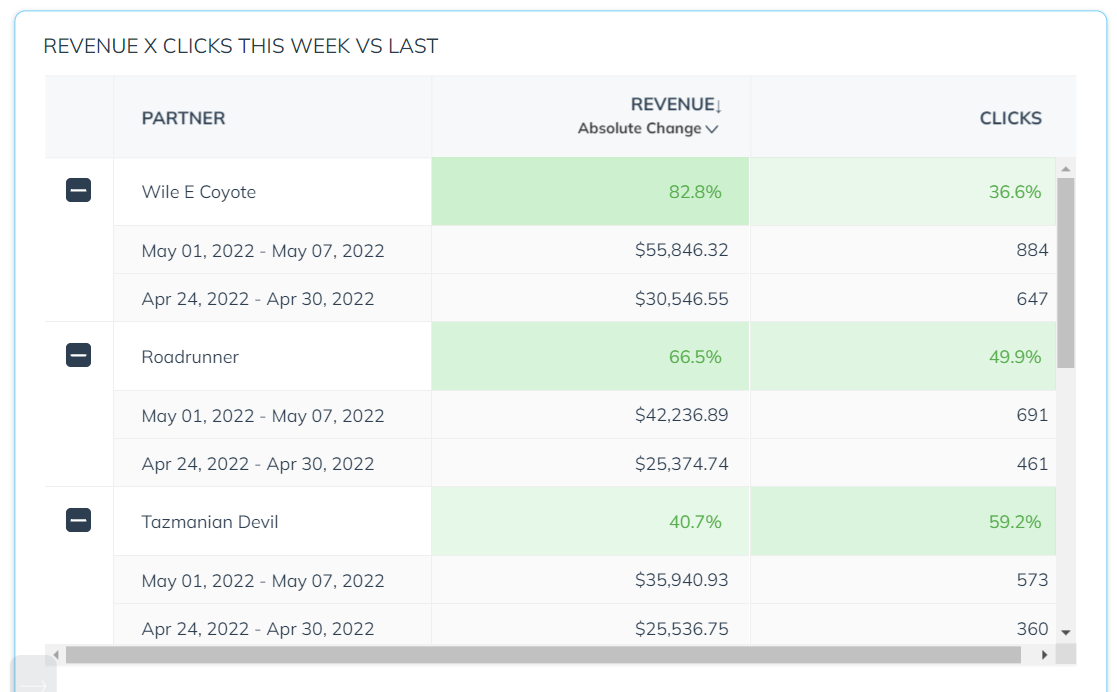Did you find it helpful? Yes No

Send feedback